# Mathematics - Signals And Systems - Exercises on Discrete-Time Fourier Series and Transform[Image1]

## Introduction

Hey it's a me again @drifter1!

Today we continue with my mathematics series about Signals and Systems in order to cover Exercises on Discrete-Time Fourier Series and Transform.

So, without further ado, let's dive straight into it!

## Discrete-Time Fourier Series Coefficients Calculation [Based on 10.3 from Ref1]

Let's consider the following periodic sequence: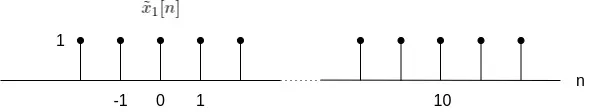Find the appropriate expression for the envelope of the Fourier series coefficients and sample it.

### Solution

In discrete-time an orthogonal pulse in the range [-N1, N1] can be represented as follows: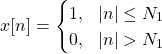Using complex exponentials, the Fourier series coefficient is thus given as follows: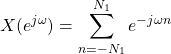As such, the samples can be taken from the sampling function, which leads us to the following envelope: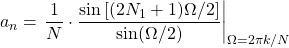In the case of this example, N1 = 2, and N = 10, and so:## Discrete-Time Fourier Transform Calculation [Based on 11.1 from Ref1]

Compute the discrete-time Fourier transform of the following signals:### Solution

The Fourier transform can be easily calculated with the help of Fourier transform pair tables and properties that we discussed throughout the series of articles.

#### a.

The first case is a slighly different representation of one of the known Fourier transform pairs, which is: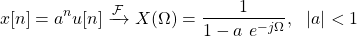For |a| > 1, this can be re-written as: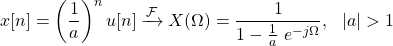Thus, for a = 6, which is the first signal, the result is: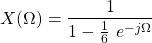#### b.

This case is quite similar to the first one. Let's start re-formulating in order to achieve such a form:From the previous example we know that:Due to linearity its possible to multiply both sides by 36, which yields: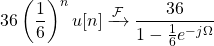From the time-shifting property, we then get the final result: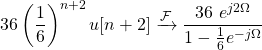#### c.

The orthogonal pulse can be easily described using unit step functions, in the following manner: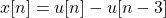The Fourier transform of the unit step function is: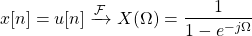And time-shifting by 3 leads to the following result: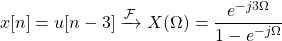So, the final result is:## Output of LTI System [Based on 11.2 from Ref1]

The following linear constant-coefficient difference equation describes an LTI system initially at rest: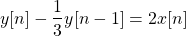Using Fourier transforms, let's evaluate y[n] for each of the following inputs:### Solution

First of all, let's take the Fourier transform of both sides of the LCCDE:As such H(Ω) is equal to: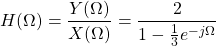#### a.

For x[n] = δ[n], X(Ω) = 1, which makes the output equal to the impulse response: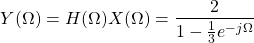and, so the Inverse Fourier Transform gives us the output y[n] and h[n] in the same time: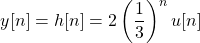#### b.

The Fourier Transform of x2[n] is:and so the output Y(Ω) is equal to:Taking the Inverse Fourier Transform gives us the final result: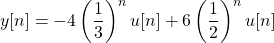## RESOURCES:

### Images

1.Block diagrams and other visualizations were made using draw.io and GeoGebra

## Final words | Next up

And this is actually it for today's post!

From next time we will start getting into concepts like Filtering, Modulation, Sampling, Interpolation etc.

See Ya!Keep on drifting!

H2
H3
H4
3 columns
2 columns
1 column
1 Comment